# Superficie Óptima de Presiones para el Diseño de Sistemas

En este trabajo se propone presentar un sistema de distribución de agua con base hidráulica (WDS) metodología de diseño llamada Superficie de Uso Óptimo de Potencia (OPUS). Su objetivo es llegar a los diseños de menor costo, la ejecución de un número reducido de iteraciones, y se centra en la creación de formas eficientes en el que la energía se disipa y el flujo se distribuye. Para su validación, el algoritmo propuesto fue probado en tres redes de referencia conocidas, publicadas con frecuencia en la literatura: de Two-Loop, Hanói y Balerma. Cuando se compara con los resultados obtenidos a través de otras metodologías, OPUS se destaca por permitir diseños con costos constructivos muy similares a los obtenidos en trabajos anteriores, pero requirió un número de iteraciones menor, especialmente para redes de tamaño real. La metodología mostró que siguiendo los principios de la hidráulica es una excelente opción para diseñar Sistema de Distribución de Agua (WDS) y también proporciona una vía alternativa al proceso de búsqueda tediosa realizada por metaheurísticos.

/var/www/pavco.com.co/public/site/pdftohtml/59a7a974b16e964cfee2ae5d7f43b703/index-html.htmlOPTIMAL POWER USE SURFACE FOR DESIGN OF WATER DISTRIBUTION

SYSTEMS

J. Saldarriaga, D. Páez, P. Cuero and N. León

1

1

Universidad de los Andes – Water Distribution and Sewer Systems Research Centre (CIACUA),

Bogotá, Colombia

ABSTRACT
This  paper  intends  to  bring  forward  a  hydraulic  based  Water  Distribution  System  (WDS)  design
methodology  named  Optimal  Power  Use  Surface  (OPUS).  Its  objective  is  to  reach  least-cost
designs, executing a reduced number of iterations, and focusing on the setting-up of efficient ways
in which energy is dissipated and flow is distributed. For its validation, the proposed algorithm was
tested  on three  well  known  benchmark  networks,  frequently  reported  in  the literature:  Two-Loop,
Hanoi and Balerma. When compared to results obtained through other methodologies, OPUS stands
out  for  allowing  designs  with  constructive  costs  very  similar  to  those  obtained  in  previous  works
but  requiring  a  number  of  iterations  several  orders  of  magnitude  bellow,  especially  for  real-size
networks.  The  methodology  proved  that  following  hydraulic  principles  is  an  excellent  choice  to
design  WDS  and  also  provides  an  alternative  path  to  the  tiresome  search  process  undertaken  by
metaheuristics.

INTRODUCTION
The optimized design of WDSs is a relevant problem at a global scale, this being due to the scarcity
of  resources  and  the  importance  of  drinking  water  for  human  life.  This  issue  aggravates  in  the
context  of  developing  countries,  where  millions  of  people  still  suffer  the  lack  of  an  adequate
service. In this context minimum-cost design methodologies are essential.

Even though the design of WDSs is supposed to consider different criteria besides the construction
costs  (e.g.  reliability,  environmental  impact  and  water  quality),  the  minimum  cost  as  the  only
objective is still used to validate and compare new design algorithms. This type of design consists
in  determining  the  diameter  size  of each pipe  of the  system  in  such a  way  that  flow  demands  are
satisfied with an adequate pressure and with a minimum capital cost. In spite of the fact that pipes
are usually manufactured in discrete-sized diameters, the amount of possible pipe configurations is
immense, which means that the problem is highly indeterminate. In fact, Yates et al. (1984) showed
that it is a NP-HARD problem and thus only approximate methods could be successful in finding

Initial approximations involved traditional optimization techniques such as enumeration, linear and
non-linear  programming.  But  more  recently  these  have  been  replaced  by  different  metaheuristic
algorithms due to their ease of implementation and other advantages like their broader search of the
solution space, a relatively small reliance on the system’s initial configuration, and their capability
of  incorporating  the  discrete-sized  diameters  restriction.  Successful  attempts  include  Genetic
Algorithms  (Savic  and  Walters,  1997),  Harmony  Search  (Geem,  2006),  Scatter  Search  (Lin  et  al.,
2007), Cross Entropy (Perelman and Ostfeld, 2007),  Simulated Annealing (Reca et al., 2007), and
Particle Swarm (Geem, 2009) among others.

These  metaheuristics  consist  in  bio-inspired  algorithms  that  randomly  generate  a  large  number  of
possible  solutions  and  test  their  fitness  in  terms  of  quality  and  capital  costs.  Generic  learning
functions are used to progressively improve the previous results. In the WDS design context, each
solution  corresponds  to  an  alternative  design,  which  means  a  different  set  of  pipe  diameter  sizes.

/var/www/pavco.com.co/public/site/pdftohtml/59a7a974b16e964cfee2ae5d7f43b703/index-html.htmlThe evaluation of each of the alternative designs requires running static hydraulic simulations, thus
a  large  number  of  iterations  is  needed  before  convergence  is  reached.  This  makes  metaheuristics
very demanding in terms of computational effort regardless their flexibility and their capability of
accomplishing  near-optimal  results.  For  this  reason,  apart  from  the  cost  of  the  final  solution,  the
number of hydraulic simulations (or iterations) is the main indicator used to measure and compare
the  efficiency  of  the  different  methodologies.  Even  though  the  learning  functions  used  in
metaheuristic  algorithms  involve  testing  the  hydraulic  performance  of  each  of  the  candidate
solutions, neither of them make use of additional hydraulic criteria.

As  a  response  to  these  tedious  algorithms,  some  researchers  have  come  through  with  new
approaches that seek to develop a hydraulic treatment of the problem. While metaheuristics intend
to optimize an objective function behaving towards the optimization variables simply as a series of
numbers  that  must  follow  certain  logic,  without  any  understanding  of  the  machinery  behind  that
logic;  these  new  approaches  try  to  characterize  the  behaviour  of  the  different  hydraulic  variables
and understand the underlying dynamics.

In  1975  I-Pai  Wu  carried  out  an  analysis  for  the  drip  irrigation  main  line  design  problem,
considering the hydraulic principles that it follows. After setting up a minimum pressure (

) at

the  end  of  the line,  still  a  big  number  of configurations  could  be  constructed.  Wu discovered  that
each  of  these  configurations  lead  to  a  different  way  in  which  energy  is  spent.  After  analysing
numerous  alternatives  he  concluded  that  the  least-cost  alternative  was  that  with  a  parabolic
hydraulic gradient line (HGL) with a sag of 15% of the total head-loss (

). Thus, optimal designs

could  be  obtained  by  computing  objective  head-loss  values  for  each  pipe  derived  from  the  HGL
fabricated using Wu´s criterion.

Later  in  1983,  Professor  Ronald  Featherstone  from  Newcastle  University  in  the  United  Kingdom
first  proposed  to  extend  Wu´s  criterion  to  the  optimization  of  looped  networks.  This  idea  seemed
like a sound possibility and was further developed by Saldarriaga (1998), who analysed hydraulic
gradient surfaces on several WDS designs obtained using metaheuristic algorithms. Based on Wu’s
criterion  and  Featherstone’s  idea,  the  works  of  Villalba  (2004)  and  Ochoa  (2009)  proved  that
hydraulic  criteria  could  be  used  as  the  basis  of  WDS  design  in  order  to  replace  the  iteration-
intensive  stochastic  approach  required  by  metaheuristics;  obtaining  promising  results,  not  only  in
performance, but also in the insight of the inner mechanics that govern WDS design.

Based  on  the  works  made  by  Ochoa  (2009)  and  Villalba  (2004),  a  first  design  methodology  was
developed  by  the  CIACUA  (Water  Distribution  and  Sewer  Systems  Research  Centre),  named
SOGH.  It was tested on three well known benchmark networks (Two-Loop,  Hanoi and Balerma).
This  paper  intends  to  bring  forward  an  improvement  to  this  WDS  design  methodology  named
Optimal Power Use Surfaces (OPUS), which proposes a net hydraulic approach following the ideas
of  the  aforementioned  authors  (Takahashi  et  al.,  2010).  The  objective  of  this  methodology  is  to
reach least-cost designs with a reduced number of iterations especially for real-size networks. This
can be accomplished through the use of deterministic hydraulic principles drawn from the analysis
of  flow  distribution  and  the  way  energy  is  used  in  the  systems.  These  principles  provide  an
alternative path to the tiresome search process undertaken by metaheuristics. Each of the steps that
make  up  the  OPUS  algorithm  are  explained  in  the  following  section.  Three  different  benchmark
problems (Two-Loop, Hanoi and Balerma) are employed to test the methodology and the results are
presented  and  discussed  in  this  paper.  Finally,  conclusions  are  drawn  from  the  outcome  obtained
and future work guidelines are stated.

METHODOLOGY
The  developed  OPUS  design  methodology  consists  in  6  basic  sub-processes  which  are  shown  in
Figure 1 and explained below in this section.

/var/www/pavco.com.co/public/site/pdftohtml/59a7a974b16e964cfee2ae5d7f43b703/index-html.html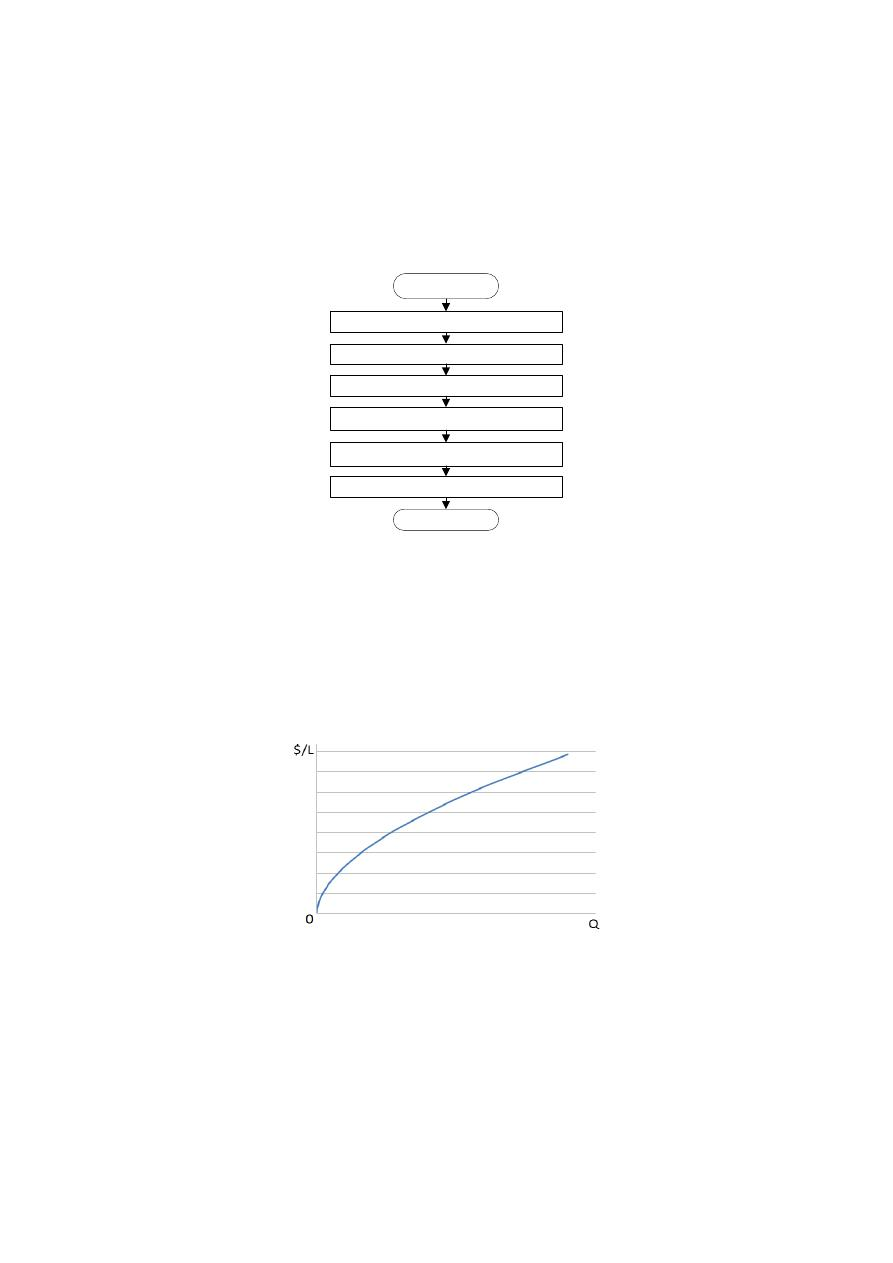Sump Search or Tree Structure. This step is based on two fundamental principles:  The first one
states that a WDS of minimum cost should convey the water to each of the demand nodes from the
water sources, through a single route. This is drawn from the fact that redundancy is hydraulically
inefficient,  even  though  it  favors  reliability.  Therefore,  open  WDSs  could  be  a  lot  cheaper  than
looped networks, reason why this sub-process intends to decompose the looped system into an open
tree-like  structure  (a  spanning  tree),  in  order  to  identify  the  nodes  in  the  original  model  that
correspond  to the  sumps  of  the  open  network  (i.e.,  nodes  with a  lower  head  than that  of  all  of  its
neighbors).

Start

Sump Search

Optimal Power Use Surface

Optimal Flow Distribution

Diameter Calculation

Diameter Round-Off

Optimization

End

Figure 1: OPUS methodology BPMN diagram.

The  second  principle  follows  from  the  flow  expression  derived  from  the  Darcy-Weisbach  and
Colebrook-White  equations.  Leaving  all  the  other  parameters  constant,  the  flow  (

)  presents  a

relation approximately proportional with the diameter to a power of 2.6. Assuming  a standard pipe
cost equation and replacing the diameter according to this proportion, the cost per length of a pipe
as a function of its design flow behaves as shown in Figure 2; which means that as the design flow
for a pipe increases, the marginal cost decreases.

Figure 2: Schematic relation between pipe cost and flow.

From  the  abovementioned  principles,  an  algorithm  was  designed  in  order  to  obtain  the  tree
structure, aggregating flow values in the least number of main routes possible. The open network is
set up starting from the water sources and then adding adjacent pipe-node pairs, one at a time. The
group  of  available  pairs  in  each  iteration  conform  the  ‘search  front’  and  each  of  these  pairs  are
assigned a cost-benefit value (

), making up a recursive process.

/var/www/pavco.com.co/public/site/pdftohtml/59a7a974b16e964cfee2ae5d7f43b703/index-html.html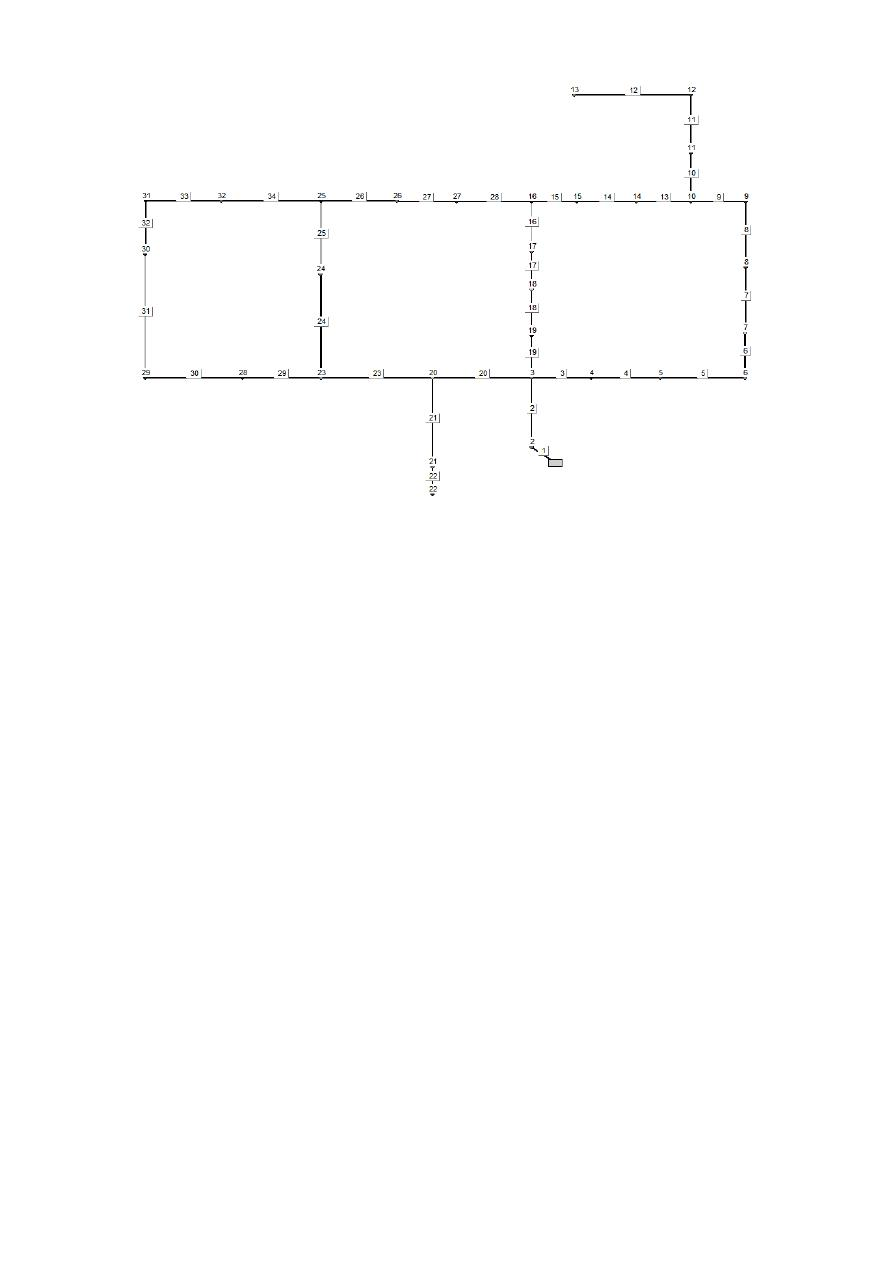Figure 3: Layout of the Hanoi WDS. The labels show pipe and nodal identification numbers.

For example, take the Hanoi benchmark WDS shown in Figure 3: Starting from the source, the first
pair to be added is the one consisting in pipe 1 and node 2 (<1, 2>). Then, the pair <2, 3> is added.
At this point the pairs <3, 4>, <19, 19> and <20, 20> can be selected. These constitute the search
front.  Figure  3  shows  the  result  for  the  entire  execution  of  the  sub-process,  where  the  pipes
highlighted (solid black) constitute the corresponding tree structure.

The  pair  in  the  front  with  the  higher  cost-benefit  value  is  selected  to  be  part  of the  tree  structure.
The cost-benefit function of a pair is calculated by computing the quotient between the demand of
the new node and the marginal cost of connecting it to  the source: This entails the addition of the
total cost of the pair’s pipe to the cost difference of transporting the additional flow through all of
the upstream pipes. It is worth noting that these are not actual costs but proportional values drawn
from the relation shown in Figure 2. The construction of the tree using this cost-benefit function has
an O(NN

2

) time complexity, where NN is the number of nodes.

The cost-benefit function is used because it favours the creation of few main routes that transport
the largest portion of the total water volume. The process concludes when all of the system nodes
have been added to the tree structure and at the end the leaf nodes in the tree structure are assigned
the status of ‘sumps’.

Optimal  Power  Use  Surface.  This  sub-process  gives  the  name  to  the  entire  methodology,  being
essential to it due to the close relation that it has with the work developed by I-Pai Wu. In this step a
set  of  objective  hydraulic  heads  (also  understood  as  objective  head losses)  is  established  for  each
pipe within the system. By analysing the behaviour of optimal designs, Ochoa (2009) corroborated
Wu’s  suggestion,  finding  that  the  optimal  hydraulic  gradient  line  for  pipe  series  was  in  effect  a
parabola.  Besides,  Ochoa  discovered  that  the  sag  of  this  parabola  depended  on  the  demand
distribution, the ratio between flow demands and pipe length, and the cost function; putting forward
a methodology for its calculation.

/var/www/pavco.com.co/public/site/pdftohtml/59a7a974b16e964cfee2ae5d7f43b703/index-html.html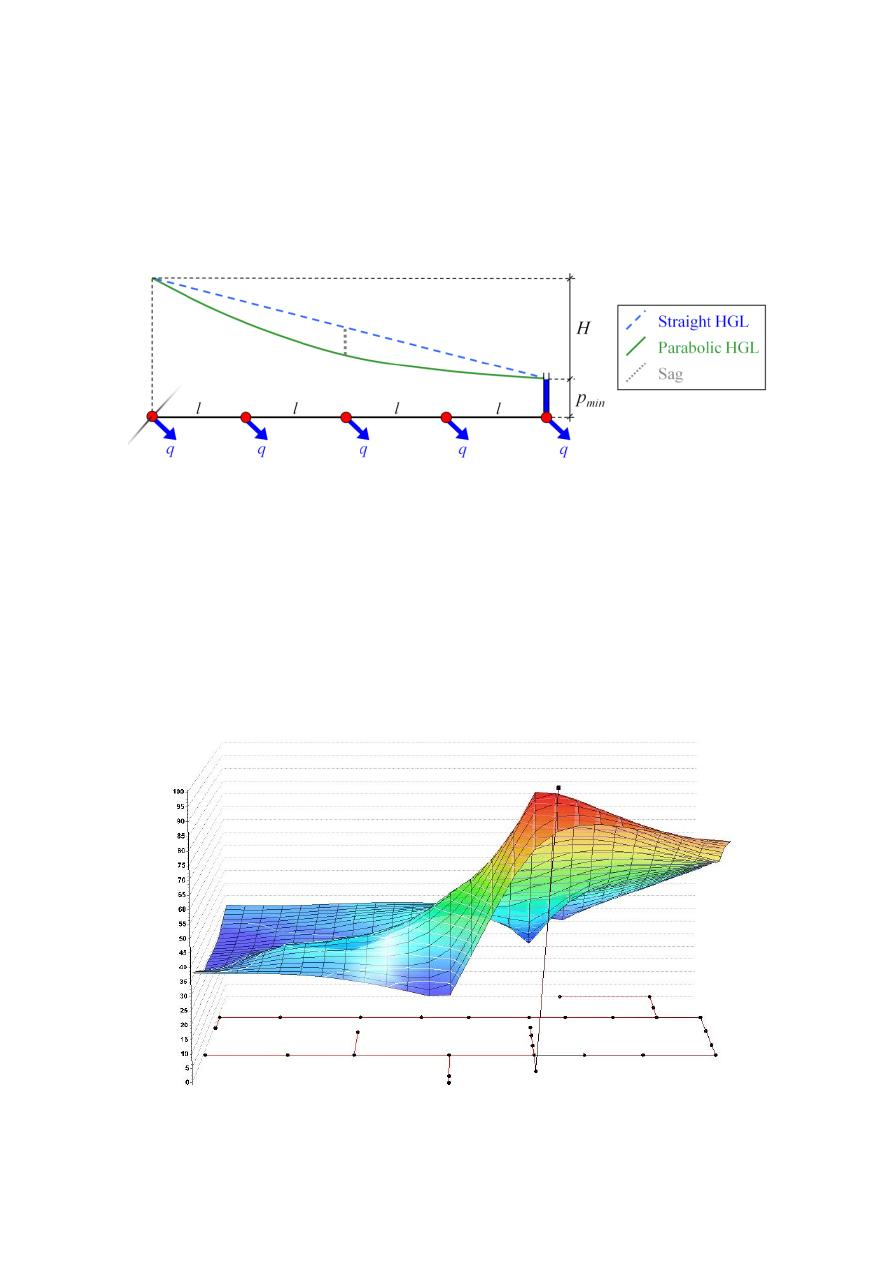Contrarily  to  the  approaches  made  by  Villalba  (2004)  and  Ochoa  (2009),  in  the  proposed
methodology,  the  optimal  power  use  surface  is  computed  in  the  tree  structure  instead  of  using  a
graph algorithm on the original network. In this sense, Ochoa’s parabolic hydraulic gradient line is
applied  to  each  of  the  branches  in  the  open  network.  First,  the  minimum  allowable  pressure  is
assigned to each of the sump nodes. Then, the topological distance for every node in the system to
its source is calculated. Knowing the head in the reservoir, the heads of the intermediate nodes in
each  branch  are  calculated  with  a  Parabolic  Head  Gradient  Line  (HGL)  as  a  function  of  their
distance to the source, as shown in Figure 4.

Figure 4: I-Pai Wu's criterion for predefining the head on each node.

As the branches converge while going over the tree upstream, it is necessary to recalculate the sag
at each intersection by weighting the flow on each downstream route. It must be taken into account
that  the  objective  parabola  has  to  be  modified  in  the  upstream  direction,  in  case  of  encountering
particularly  elevated  nodes,  to  make  sure  that  the  assigned  head  values  meet  the  pressure
requirements  in  every  instance.  Once  this  sub-process  is  concluded,  all  nodes  must  have  an
objective head value assigned, thus a flow is required in order to calculate the diameter of each pipe
in the network.

Figure 5 shows an example of the optimal power use surface for the Hanoi network. Note that for
the water source the HGL corresponds to the total head available in the reservoir and for the sump
nodes it was assigned the minimum pressure.

Figure 5: Assigned surface for the Hanoi network.

H

GL

(m

/var/www/pavco.com.co/public/site/pdftohtml/59a7a974b16e964cfee2ae5d7f43b703/index-html.html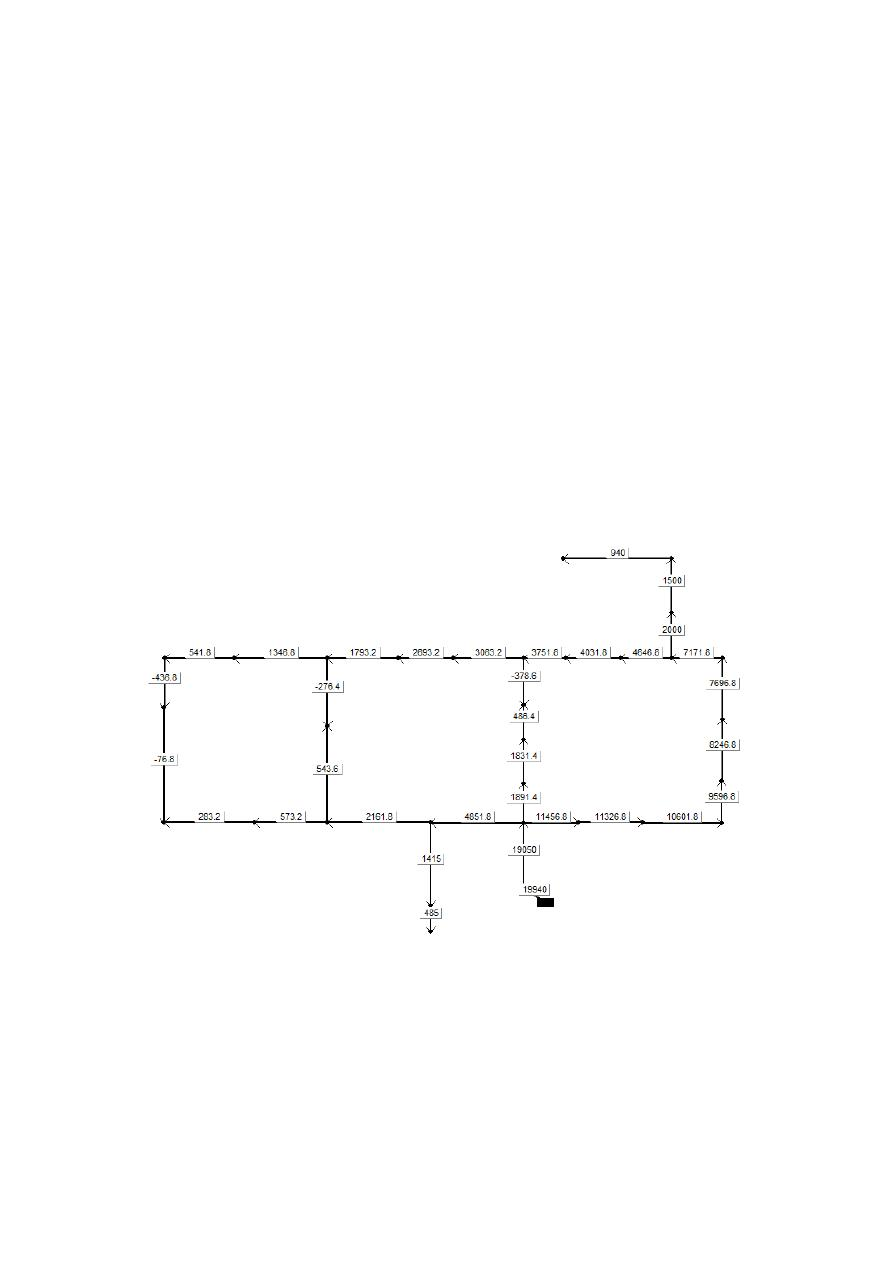Optimal  Flow  Distribution.  This  step  assigns  a  design  flow  to  every  pipe  in  the  system.
Considering that in a looped network a specific hydraulic gradient surface could be obtained by an
infinite number of continuous diameter configurations (Saldarriaga et al., 2011), it is necessary to
predefine an  objective  flow  for  each  pipe  in  order  to  obtain a  configuration  that minimizes  costs.
Therefore,  this  sub-process  pretends  to  find  a  unique  flow  distribution  scheme  that  respects  mass
conservation and conforms to the optimal power use surface previously obtained. In this case, the
process is executed using the original graph instead of the spanning tree.

Starting  with  the  sumps,  the  flow  demand  is  divided  into  the  upstream  pipes  according  to  the
following criterion: Only one pipe will be assigned the largest flow value, while the others will be
assigned the flow that corresponds to the minimum diameter available (

. In order to determine

the principal pipe (i.e. the pipe that will have the largest portion of the total flow demanded), several
criterions can be used to evaluate their fitness. Same as in the tree structure step, the function

is  used,  which  means  that  the  pipe  with  the  biggest  value  of  this  function  will  be  defined  as  the
principal pipe. For non-sump nodes, the total demand is calculated adding its own flow demand and
the flow demanded downstream. An iterative-recursive algorithm (IRA) can be used to perform all
of the calculations with an O(NN) time complexity. At the end of the process, all of the pipes in the
system must have been assigned an objective flow value. Note that this step has a higher impact in
the case of looped systems.

As an example, Figure 6 shows the optimal flow distribution that corresponds to the Hanoi network.

Figure 6: Optimal flow distribution for the Hanoi network (Flow rates in m

3

/h).

Diameter  Calculation.  This  sub-process  assigns  continuous  diameter  sizes  to  all  pipes.  Having
predefined  the  objective  head  losses  and  the  design  flow  rate  for  each  pipe  in  the  system,  the
continuous  diameter  needed  is  given  by  a  straightforward  calculation.  This  calculation  is  explicit
when  the  Hazen-Williams  equation  is  used  and  iteratively  for  the  Darcy-Weisbach  and  the
Colebrook-White  equations.  The  resulting  continuous  design  is  in  theory  a  full-operational  WDS,
with a cost very close to the minimum. Due to the limited availability of diameter sizes, a next step
is required to transform this “optimal” design to a feasible one.

/var/www/pavco.com.co/public/site/pdftohtml/59a7a974b16e964cfee2ae5d7f43b703/index-html.htmlDiameter Round-Off. This step consists in approximating each continuous diameter to a discrete
value  from  the  list  of  commercially  available  diameter  sizes,  which  is  represented  by  the  set
{

}. It was found that rounding to the nearest equivalent flow value offers the best

results,  even  though  it  can  be  done  following  several  criterions.  This  is  done  by  elevating  the
diameter values to a power of 2.6, as explained in the Tree Structure step. Unfortunately, this step
affects drastically the system’s hydraulic behaviour, especially if all the diameter sizes are rounded
up or down.

Optimization
This  final  sub-process  has  two  main  goals:  The  first  one  is  to  ensure  every  node  has  a  pressure
higher than or equal to

; secondly, it seeks for possible cost reductions. Several criteria could be

used to establish the order in which pipes diameter values must be increased. It was found that the
pipes  with  larger  unit  head-loss  difference  between  real  and  objective  values  should  be  changed
first. The process must continue until the whole system has acceptable pressures. The second part
executes a two-way sweep starting from the reservoirs going towards the sumps in the direction of
the flow, and then backwards: The reduction of each pipe’s diameter is considered twice. If any of
these  changes  entails  a  pressure  deficit  it  must  be  reversed  immediately,  otherwise  it  holds.  To
make sure minimum pressure is not being violated numerous hydraulic simulations are required.

In  first  place,  the  diameter  size  of  one  pipe  is  increased  iteratively  while  there  are  nodes  with
pressure  deficit.  Thus,  this  sub-process  requires  the  most  number  of  iteration  of  the  whole
methodology,  being  necessary  to  run  a  hydraulic  simulation  per  pipe,  for  each  single  diameter
modification.  This  sole  heuristic  can  be  used  alone  to  obtain  sound  designs,  in  spite  of  this,  it  is
strongly dependant on the initial pipe configuration.

RESULTS
The  OPUS  methodology  was  used  on  three  benchmark  systems:  Two-Loop,  Hanoi  and  Balerma.
Different  configurations  of  the  parameters  defined  on  each  step  of  the  methodology  were  tested,
looking  for  optimal  designs  with  discrete  diameters  (

{

}  contains  only  diameter

available  at  the  local  market)  and,  in  some  cases,  continuous  ones  (

)  as  potentially  near

optimal starting designs for the Round-off and Optimization sub-processes.

Two-Loop
Detailed  information about this  WDS  can  be  found  in  Alperovits  and  Shamir  (1977).  The  Hazen-
Williams  head-loss  equation  was  used  with  a  roughness  coefficient

,  as  specified  in  the

mentioned publication, and also the unit prices table for each diameter size was adopted. With these
values  and  with  a  minimum  allowable  pressure  for  every  node  of  30  m,  the  WDS  was  designed
using the SOGH algorithm which is the OPUS predecessor methodology.

The optimal discrete design was reached after 51 hydraulic simulations which leaded to a \$419,000
network. The number of hydraulic simulations reported by other authors who also reached this cost
is shown in Table 1.

Table 1: Reported number of iterations before reaching a cost of \$419.000 for the Two-Loop WDS.

Algorithm

Number of iterations

Genetic algorithms (Savic & Waters, 1997)

65,000

Simulated annealing (Cunha & Sousa, 1999)

25,000

Genetic algorithms (Wu & Simpson, 2001)

7,467

Shuffled frog leaping (Eusuff & Lansey, 2003)

11,155

/var/www/pavco.com.co/public/site/pdftohtml/59a7a974b16e964cfee2ae5d7f43b703/index-html.html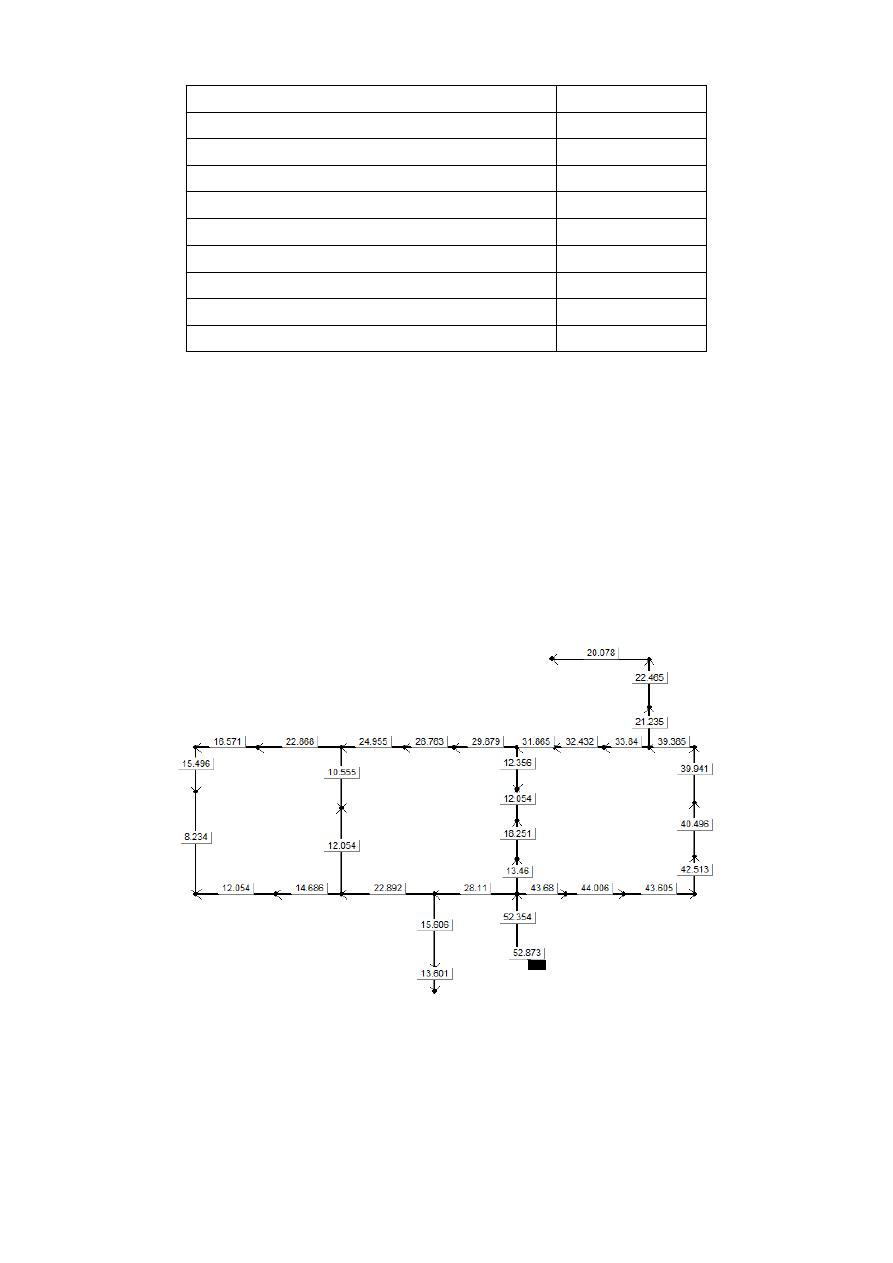Shuffled complex evolution (Liong & Atiquzzaman, 2004)

1,019

Genetic algorithms (Reca & Martínez, 2006)

10,000

Particle swarm optimization (Suribabu, 2006)

5,138

Harmony search (Geem, 2006)

1,121

Cross entropy (Perelman & Ostfeld, 2007)

35,000

Scatter search (Lin et al., 2007)

3,215

Particle swarm harmony search (Geem, 2009)

204

Differential evolution (Suribabu C. , 2010)

4,750

Honey-bee mating optimization (Mohan, 2010)

1,293

SOGH (Ochoa, 2009)

51

Hanoi
The Hanoi network was first presented by  Fujiwara and Khang (1990) and similarly to Two-Loop
network, it has become a well-known benchmark WDS. The head-loss equation commonly used is
Hazen-Williams  with  a

,  the minimum  pressure  for  the  design  scenario is  30  m and  the

pipes’ costs can be calculated using a potential function of the diameter with a unit coefficient of
\$1.1/m and an exponent of 1.5.

The least cost continuous design reached for Hanoi presents a cost of  \$5,456,806, and is shown in
Figure  7.  It  is  worth  noting  that  the  continuous  design  involves  diameter  values  higher  than  40
inches,  which  corresponds  to  the  maximum  allowable  diameter  according  to  Fujiwara  and  Khang
(1990). Therefore, two different discrete designs were performed: The first one being based on the
original diameter list, and the second one considering the availability of a 50 inches diameter.

Figure 7: Hanoi network least cost continuous design (diameters in inches).

The  OPUS  methodology  for  the  first  case  reached  a  cost  of  \$6’147,882.45  after  83  iterations.
Although this is not the least cost reported, the number of hydraulic simulations needed to reach this
result is three orders of magnitude smaller than that of other approaches, as can be seen in  Table 2
The pipe diameter sizes in inches for this configuration are: 40, 40, 40, 40, 40, 40, 40, 40, 40, 30,
24, 24, 16, 16, 12, 12, 20, 20, 24, 40, 20, 12, 40, 30, 30, 20, 16, 12, 16, 12, 12, 12, 16 and 30 (these
diameters are shown in order of pipe identification number).

/var/www/pavco.com.co/public/site/pdftohtml/59a7a974b16e964cfee2ae5d7f43b703/index-html.htmlTable 2: Reported costs and number of iterations for the Hanoi WDS.

Algorithm

Cost (millions)

Number of iterations

Genetic Algorithm (Savic and Walters, 1997)

\$6.073

1,000,000

Simulated annealing (Cunha and Sousa, 1999)

\$6.056

53,000

Harmony search (Geem, 2002)

\$6.056

200,000

Shuffled frog leaping (Eusuff and Lansey, 2003)

\$6.073

26,987

Shuffled complex evolution (Liong & Atiquzzaman, 2004)

\$6.220

25,402

Genetic Algorithm (Vairavamoorthy, 2005)

\$6.056

18,300

Ant colony optimization (Zecchin et al., 2006)

\$6.134

35,433

Genetic Algorithms (Reca & Martínez, 2006)

\$6.081

50,000

Genetic Algorithms (Reca et al., 2007)

\$6.173

26,457

Simulated annealing (Reca et al., 2007)

\$6.333

26,457

Simulated annealing with tabu search (Reca et al., 2007)

\$6.353

26,457

Local search with simulated annealing (Reca et al., 2007)

\$6.308

26,457

Harmony search (Geem, 2006)

\$6.081

27,721

Cross entropy (Perelman & Ostfeld, 2007)

\$6.081

97,000

Scatter search (Lin et al., 2007)

\$6.081

43,149

\$6.056

18,000

\$6.190

18,000

Particle swarm harmony search (Geem, 2009)

\$6.081

17,980

Heuristic based approach (Mohan S. a., 2009)

\$6.701

70

Differential evolution (Suribabu C. , 2010)

\$6.081

48,724

Honey-bee mating optimization (Mohan, 2010)

\$6.117

15,955

Heuristic based approach (Suribabu C. , 2012)

\$6.232

259

SOGH (Ochoa, 2009)

\$6.337

94

Optimal power use surface (this study)

\$6.173

83

Extrapolating the cost function for a  50” diameter it would have a unit  cost of \$388.91/m. Taking
this into account, the total cost of the design obtained following the OPUS algorithm was of only
\$5’342,840.13, as the real hydraulic gradient surface resembled the optimal in a higher degree. The
diameter sizes in inches are: 50, 50, 40, 40, 40, 40, 40, 24, 24, 40, 20, 20, 12, 12, 12, 16, 20, 20, 20,
40, 16, 12, 40, 30, 24, 20, 12, 12, 12, 12, 12, 12, 12 and 20.

Balerma
Balerma corresponds to a WDS of an irrigation district in Almería, Spain. The pipe diameter sizes
commercially  available  for  its  design  are  manufactured  exclusively  in  PVC,  with  an  absolute
roughness  coefficient  of  0.0025  mm.  The  minimum  pressure  allowable  is  of  20  m  and  the  pipes’
costs  are  calculated  using  a  potential  function,  with  a  power  of  2.06.  Its  topology  is  presented  in
Figure 8.

/var/www/pavco.com.co/public/site/pdftohtml/59a7a974b16e964cfee2ae5d7f43b703/index-html.html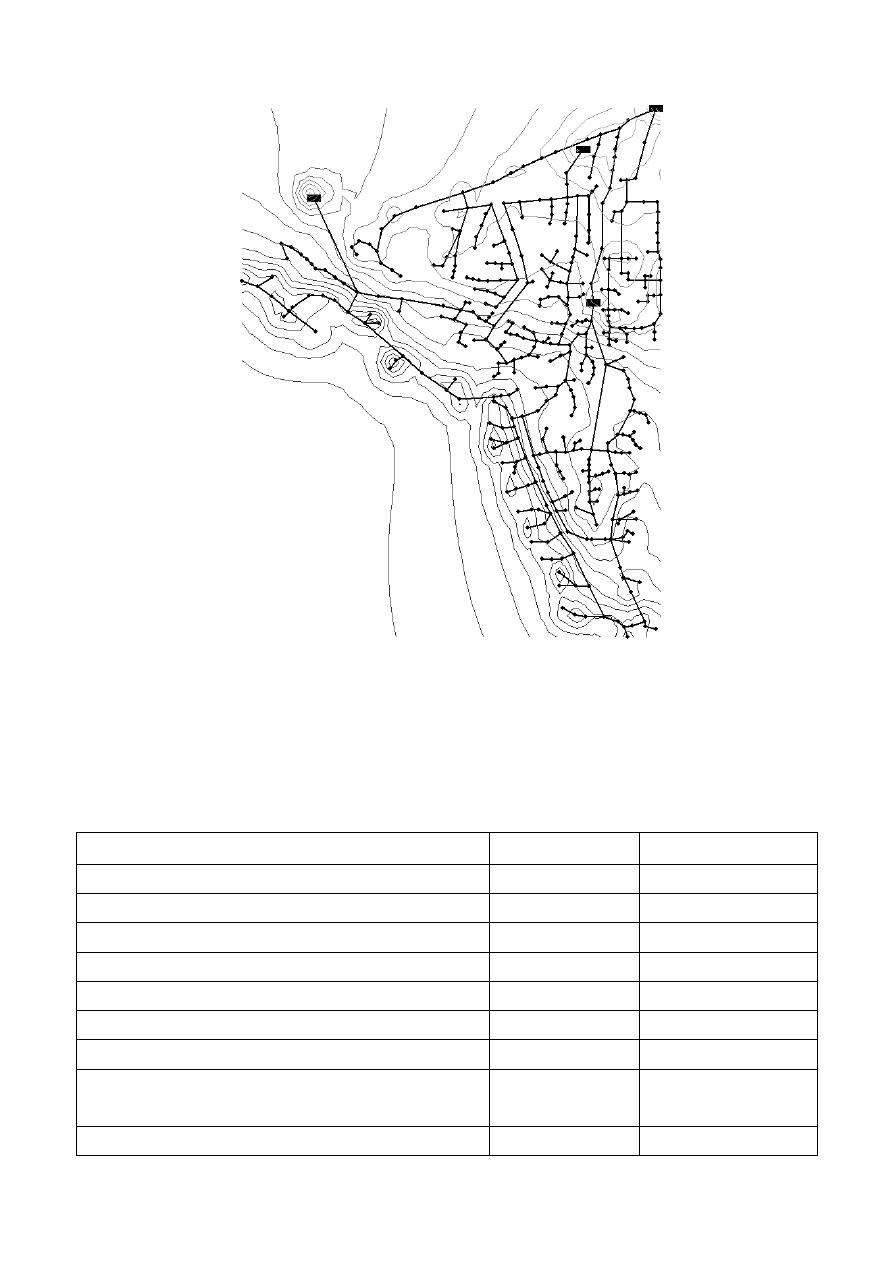Figure 8: Topology of the Balerma network.

As a result of implementing the OPUS methodology on this network, a €1.755 millions continuous
design  was  founded.  Also,  after  executing  the  round-off  and  optimization  processes,  the  optimal
discrete design was reached requiring 957 hydraulic simulations which leaded to a €2.106 millions
network. Table 3 presents other reported costs and their respective number of iterations.

Table 3: Reported costs and number of iterations for the Balerma WDS.

Algorithm

Cost (

€

millions)  Number of iterations

Genetic algorithm (Reca & Martínez, 2006)

2.302

10.000.000

Harmony search (Geem, 2006)

2.601

45.400

Harmony search (Geem, 2006)

2.018

10.000.000

Genetic algorithm (Reca et al., 2007)

3.738

45.400

Simulated annealing (Reca et al., 2007)

3.476

45.400

Simulated annealing with taboo search (Reca et al., 2007)

3.298

45.400

Local search with simulated annealing (Reca et al., 2007)

4.310

45.400

Hybrid discrete dynamically dimensioned search

(Tolson, 2009)

1,940

30,000,000

Harmony search with particle swarm (Geem, 2009)

2.633

45.400

/var/www/pavco.com.co/public/site/pdftohtml/59a7a974b16e964cfee2ae5d7f43b703/index-html.htmlSOGH (Ochoa, 2009)

2.100

1.779

Memetic algorithm (Baños, 2010)

3,120

45,400

Genetic heritage evolution by stochastic transmission

(Bolognesi, 2010)

2,002

250,000

Differential evolution (Zheng, 2012)

1,998

2,400,000

1,983

1,300,000

Optimal power use surface (this study)

2.106

957

CONCLUSIONS
The  WDS  least-cost  design  methodology  known  as  Optimal  Power  Use  Surface  (OPUS)  herein
introduced,  considers  optimal  distribution  pattern  of  flow  in  the  network  as  a  way  of  spending
energy  properly.  This  approach  differentiates  it  from  metaheuristic  algorithms  that  explore  the
solution space without considering hydraulic principles.

The methodology significantly reduces the number of iterations and keeps the constructive costs of
the network significantly close to the minimum. In the case of Hanoi the difference results only of
1.9% with respect to the lowest cost reported in the literature and with a number of iterations three
orders of magnitude below.

OPUS  is  a  methodology  that  worked  well  on  large  networks,  making  it  possible  to  minimize
constructive costs in a very reduced number of iterations. On the other hand, when applied to small
WDSs, the hydraulics result very affected due to the relative difference between the tree structure
and  the  real  looped  network.  Taking  into  account  that  the  design  is  executed  based  on  the  open
network (spanning tree), the final result ends up being governed by the optimization process and not
by the steps based on hydraulic principles.

This methodology clearly proves that considering hydraulic bases allows the optimization of WDS
design  to  reduce  significantly  the  number  of  iterations  required.  Theoretical  networks  with  some
restrictions  that  limit  the  design  possibilities  were  tested  in  this  paper.  For  this  reason,  it  is
recommended  to  test  this  methodology  on  real  networks  and  including  different  additional
objectives  such  as  maximizing  reliability  and  minimizing  leakage.  Finally  energy  approaches  can
also be applied to calibrate models and design system operation.

REFERENCES

Alperovits,  E.,  and  Shamir,  U.  (1977)  Design  of  optimal  water  distribution  systems.  Water

Resources Research, Vol.13, No.6, pp. 885-900.

Baños,  C.  G.  (2010).  A  memetic  algorithm  applied  to  the  design  of  water  distribution  networks.

Applied Soft Computing, 261-266.

Bolognesi,  A.  e.  (2010).  Genetic  Heritage  Evolution  by  Stochastic  Transmission  in  the  optimal

design of water distribution networks. Advances in Engineering Software, 792-801.

Cunha,  M.  a.  (1999).  Water  distribution  network  design  optimization:  Simulated  annealing

approach. J. Water Resour. Plan. Manage. , 215-221.

Eusuff,  M.  a.  (2003).  Optimization  of  water  distribution  network  design  using  the  shuffled  frog

leaping algorithm. J. Water Resour. Plan. Manage. , 210-225.

Fujiwara,  O.  and  Khang,  D.  (1990).  A  two  phase  decomposition  methods  for  optimal  design  of

looped water distribution networks. Water Resources Research, Vol.26, No. 4, pp. 539-549.

Geem,  Z.  K.  (2002).  Harmony  search  optimization:  Application  to  pipe  network  design.  Int.  J.

Model. Simulat. , 125-133.

/var/www/pavco.com.co/public/site/pdftohtml/59a7a974b16e964cfee2ae5d7f43b703/index-html.htmlGeem,  Z.  K.  (2006).  Optimal  cost  design  of  water  distribution  networks  using  harmony  search.

Engineering Optimization, Vol.38, No.3, pp. 259-277.

Geem,  Z.  K.  (2009).  Particle-swarm  harmony  search  for  water  network  design.  Engineering

Optimization, Vol.41, No.4, pp. 297-311.

Kadu,  M.  R.  (2008).  Optimal  design  of  water  networks  using  a  modified  genetic  algorithm  with

reduction in search space. J. Water Resour. Plan. Manage. , 147-160.

Lin,  M.,  Liu,  G.  and  Chu,  C.,  (2007)  Scatter  search  heuristic  for  least-cost  design  of  water

distribution networks, Engineering Optimization, Vol.39, No.7, pp. 857-876.

Liong, S. and Atiquzzaman, M. (2004) Optimal design of water distribution network using shuffled

complex evolution. Journal of the Institution of Engineers, Vol.44, No.1, pp. 93-107.

Mohan,  S.  a.  (2010).  Optimal  water  distribution  network  design  with  Honey-Bee  mating

optimization. J. Water Resour. Plan. Manage., 117-126.

Mohan,  S.  a.  (2009).  Water  distribution  network  design  using  heuristics-based  algorithm.  J.

Comput.Civ. Eng., 249-257.

Ochoa,  S.  (2009).  Optimal  design  of  water  distribution  systems  based  on  the  optimal  hydraulic

gradient  surface  concept.  MSc  Thesis,  dept.  of  Civil  and  Environmental  Engineering,
Universidad de los Andes, Bogotá, Col. (In Spanish).

Perelman,  L.  and  Ostfeld,  A.  (2007).  An  adaptive  heuristic  cross  entropy  algorithm  for  optimal

design of water distribution systems. Engineering Optimization, Vol.39, No.4, pp. 413-428.

Reca,  J.  and  Martínez,  J.  (2006).  Genetic  algorithms  for  the  design  of  looped  irrigation  water

distribution networks. Water Resources Research, Vol.44, W05416.

Reca,  J.,  Martínez,  J.,  Gil,  C.  and  Baños,  R.  (2007).  Application  of  several  meta-heuristic

techniques  to  the  optimization  of  real  looped  water  distribution  networks.  Water  Resources
Management
, Vol.22, No.10, pp. 1367-1379.

Saldarriaga, J.  (1998 and  2007).  Hidráulica  de  Tuberías. Abastecimiento  de Agua,  Redes, Riegos

Ed. Alfaomega. Ed. Uniandes. ISBN: 978-958-682-680-8.

Saldarriaga,  J.,  Takahashi,  S.,  Hernández,  F.  and  Escovar,  M.  (2011).  Predetermining  pressure

surfaces in water distribution system design. In Proceedings of the World Environmental and
Water Resources Congress 2011, ASCE

Savic,  D.  and  Walters,  G.  (1997).  Genetic  algorithms  for  least  cost  design  of  water  distribution

networks. J. Water Resour. Plan. Manage., 67-77.

Suribabu,  C.  a.  (2006).  Design  of  water  distribution  networks  using  particle  swarm  optimization.

Urban Water Journal, 111-120.

Suribabu,  C.  (2010).  Differential  evolution  algorithm  for  optimal  design  of  water  distribution

networks. J. of Hydroinf., 66-82.

Suribabu, C. (2012).  Heuristic Based Pipe Dimensioning Model for Water Distribution Networks.

Journal of Pipeline Systems Engineering and Practice, 45 p.

Takahashi,  S.,  Saldarriaga,  S.,  Hernández,  F.,  Díaz,  D.  and  Ochoa,  S.  (2010).  An  energy

methodology  for  the  design  of  water  distribution  systems.  In  Proceedings  of  the  World
Environmental and Water Resources Congress 2010, ASCE
.

Tolson,  B.  A.  (2009).  Hybrid  discrete  dynamically  dimensioned  search  (HD-DDS)  algorithm  for

water distribution system design optimization. Water Resources Research, 45 p.

Vairavamoorthy,  K.  a.  (2005).  Pipe  index  vector:  A  method  to  improve  genetic-algorithm-based

pipe optimization. J. Hydraul. Eng., 1117-1125.

Villalba,  G.  (2004).  Optimal  combinatory  algorithms  applied  to  the  design  of  water  distribution

systems.  MSc  Thesis,  dept.  of  Systems  and  Computation  Engineering,  Universidad  de  los
Andes.

Wu,  I.  (1975).  Design  of  drip  irrigation  main  lines.  Journal  of  Irrigation  and  Drainage  Division

Vol.101, No.4, pp. 265-278.

Wu, Z. and Simpson, A. (2001). Competent genetic evolutionary optimization of water distribution

systems. Journal of computing in civil engineering. Vol.15, No.2, 2001, pp. 89-101.

/var/www/pavco.com.co/public/site/pdftohtml/59a7a974b16e964cfee2ae5d7f43b703/index-html.htmlYates, D., Templeman, A. and Boffey, T. (1984). The computational complexity of the problem of

determining  least capital  cost  designs  for  water  supply  networks.  Engineering  Optimization
Vol. 7, No.2, pp. 142-155.

Zecchin,  A.,  Simpson,  A.,  Maier,  H.,  Leonard,  M.,  Roberts,  A.,  and  Berrisfors,  M.  (2006)

Application  of  two  ant  colony  optimization  algorithms  to  water  distribution  system
optimization. Mathematical and Computer Modeling, Vol.44, No. 5-6, pp. 451-468.

Zheng,  F.  e.  (2012).  A  Self

‐Adaptive  Differential  Evolution  Algorithm  Applied  to  Water

Distribution System Optimization. Journal of Computing in Civil Engineering, 45 p.